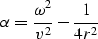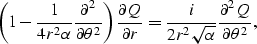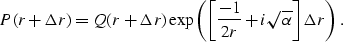# Short Note Errors in SEP-81

Dave Nichols

nichols@gatwick.Geco-Prakla.slb.com

There were several errors in the equations in my thesis. They were caused by some careless cutting and pasting from the wrong version of a paper and some even more careless proofreading on my part. I would like to thank Stew Levin and Paul Fowler for pointing out these problems.

There errors are in the derivation of a paraxial extrapolation operator for the wave equation in polar coordinates on page 20. Fortunately the code that was used in my thesis and distributed on the CD version is correct. It implements the equations described here not the ones in my thesis.

PARAXIAL APPROXIMATIONS IN POLAR COORDINATES

When the velocity is a function of radius and angle, the simple Fourier domain methods are no longer valid. However, as in the more familiar rectangular-coordinate formulations, the Helmholtz equation can be approximated by a paraxial wave equation:(1)
where,(2)
After splitting this equation becomes(3)
followed by,(4)

Discretization of the first equation yields a tridiagonal set of equations to be solved for each radial step. The implementation of this scheme is almost identical to a standard 45-degree implicit extrapolator in rectangular coordinates. We only need to change the calculation of the coefficients of the tridiagonal system and the exponent of phase shift.